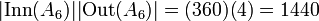# Difference between revisions of "Automorphism group of alternating group:A6"

View a complete list of particular groups (this is a very huge list!)[SHOW MORE]

## Definition

This group is defined in the following equivalent ways:

1. It is the automorphism group of alternating group:A6.
2. It is the automorphism group of symmetric group:S6.
3. It is the projective semilinear group of degree two over the field of nine elements, i.e., it is the group$P\Gamma L(2,9)$.

Note that for any$n \ne 2,3,6$, the automorphism group of the alternating group$A_n$ is precisely the symmetric group$S_n$, which is a complete group. The case$n = 2$ is uninteresting. For$n = 3$, the automorphism group of$A_n$ is$\mathbb{Z}/2\mathbb{Z}$, the cyclic group of order two. The case$n = 6$ is the only case where the automorphism group of the alternating group is strictly bigger than the symmetric group. Similarly, it is the only case where the automorphism group of the symmetric group is strictly bigger than the symmetric group. Further information: symmetric groups on finite sets are complete

## Arithmetic functions

Want to compare and contrast arithmetic function values with other groups of the same order? Check out groups of order 1440#Arithmetic functions

### Basic arithmetic functions

Function Value Similar groups Explanation for function value
order (number of elements, equivalently, cardinality or size of underlying set) 1440 groups with same order As$\operatorname{Aut}(A_6)$:$|\operatorname{Inn}(A_6)||\operatorname{Out}(A_6)| = (360)(4) = 1440$
As$\operatorname{Aut}(S_6)$:$|\operatorname{Inn}(S_6)||\operatorname{Out}(S_6)| = (720)(2) = 1440$
As$P\Gamma L(2,q), q = p^r, q = 9, p = 3, r = 2$:$r(q^3 - q) = 2(9^3 - 9) = 1440$

### Arithmetic functions of a counting nature

Function Value Similar groups Explanation for function value
number of conjugacy classes 13 groups with same order and number of conjugacy classes | groups with same number of conjugacy classes As$P\Gamma L(2,p^2), p = 3$ (odd):$(p^2 + 3p + 8)/2 = (3^2 + 3 \cdot 3 + 8)/2 = 13$ (more here)

## GAP implementation

### Group ID

This finite group has order 1440 and has ID 5841 among the groups of order 1440 in GAP's SmallGroup library. For context, there are 5,958 groups of order 1440. It can thus be defined using GAP's SmallGroup function as:

SmallGroup(1440,5841)

For instance, we can use the following assignment in GAP to create the group and name it$G$:

gap> G := SmallGroup(1440,5841);

Conversely, to check whether a given group$G$ is in fact the group we want, we can use GAP's IdGroup function:

IdGroup(G) = [1440,5841]

or just do:

IdGroup(G)

to have GAP output the group ID, that we can then compare to what we want.

### Other descriptions

Description Functions used
AutomorphismGroup(AlternatingGroup(6)) AutomorphismGroup, AlternatingGroup
AutomorphismGroup(SymmetricGroup(6)) AutomorphismGroup, SymmetricGroup